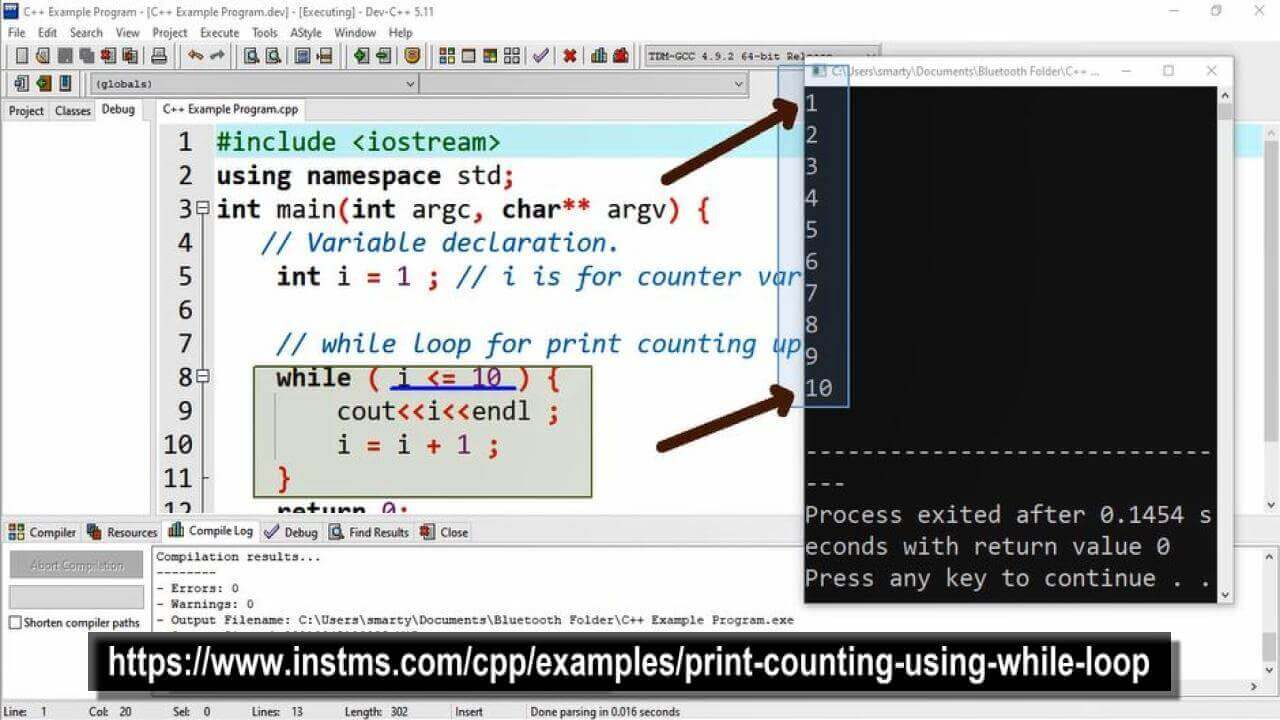# Print Counting Using While Loop in CPP

## Source Code

#include <iostream>

using namespace std;

int main(int argc, char** argv) {

// Variable declaration.
int i = 1 ; // i is for counter variable.

// while loop for print counting upto 10
while ( i <= 10 ) {
cout<<i<<endl ;
i = i + 1 ;
}
return 0;
}

##Working:

In this program example we use while loop and test using Dev-C++. For print counting using loop statement we are required a counter variable, so that here a variable named i is declared.

We have initialized this variable with 1 (one) and in while condition we set upper limit (condition) as less than equal to 10. It means that this loop will iterate its statements 10 times. Each and every time this will increment value of counter variable by 1.